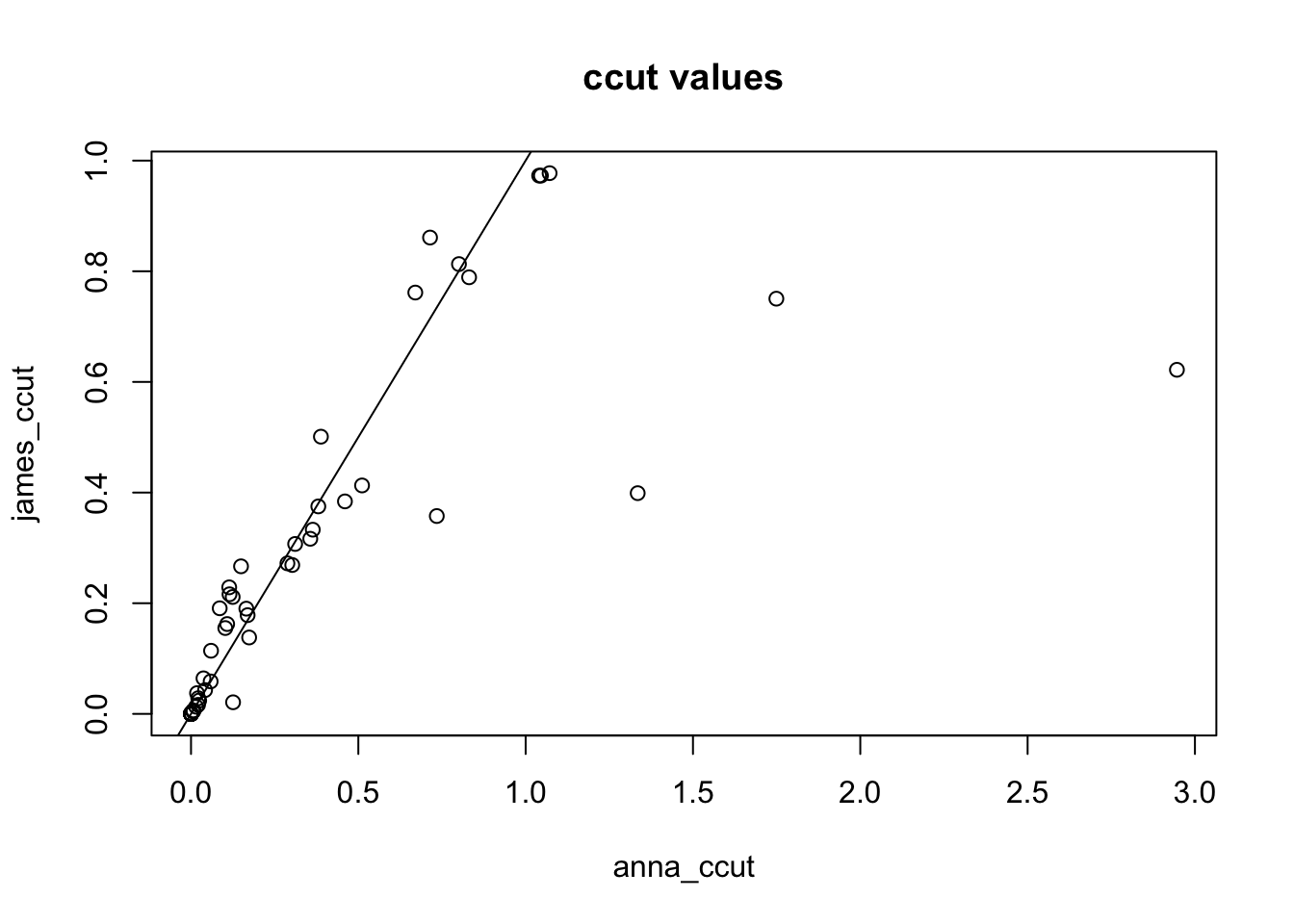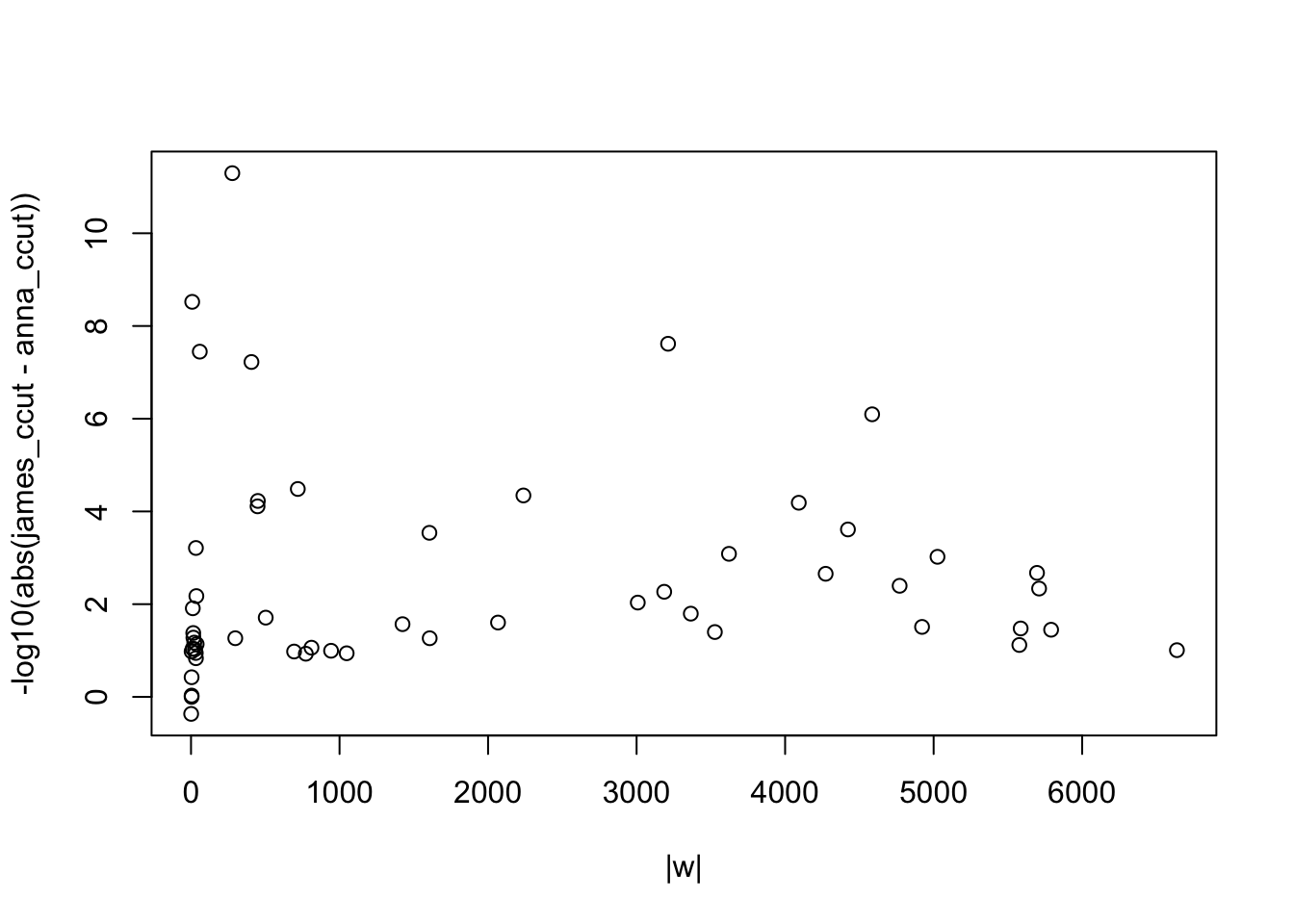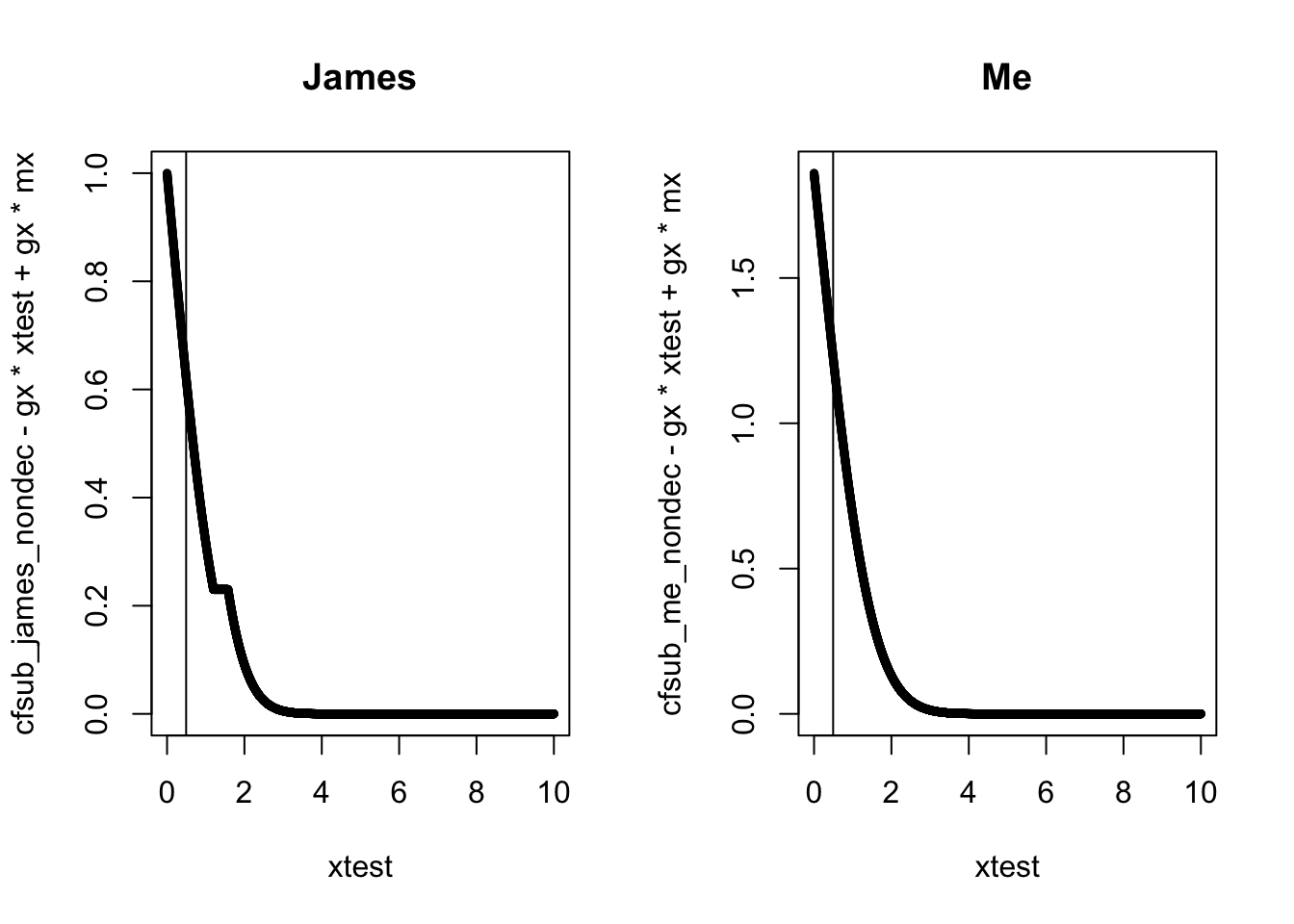### Extra functions

#' zq_h0_func
#'
#' function to estimate P(ZQ>zq|H_0^p) using a GMM fitted to ZQ|P>1/2
#'
#' @param zq
#' @param dens output from densityMclust() fitting a GMM to ZQ|P>1/2
#'
#' @return Estimate of P(ZQ>zq|H_0^p)
#' @export
#'
zq_h0_func <- function(zq, dens) {
sum <- 0
for(i in 1:length(dens$parameters$mean)){
sum <- sum + dens$parameters$pro[i] * pnorm(zq,mean = dens$parameters$mean[i], sd = sqrt(dens$parameters$variance$sigmasq[i]), lower.tail = FALSE) } return(sum) } #' q_h0_func #' #' function to estimate P(Q<q|H_0^p) using a GMM fitted to Q|P>1/2 #' #' @param q #' @param dens output from densityMclust() fitting a GMM to Q|P>1/2 #' #' @return Estimate of P(Q<q|H_0^p) #' @export #' q_h0_func <- function(q, dens) { sum <- 0 for(i in 1:length(dens$parameters$mean)){ sum <- sum + dens$parameters$pro[i] * pnorm(q,mean = dens$parameters$mean[i], sd = sqrt(dens$parameters$variance$sigmasq[i]), lower.tail = FALSE)
}
return(sum)
}

#' joint_func_zq
#'
#' function to estimate P(ZP>zp, ZQ>zq) using a bivariate GMM fitted to ZP, ZQ
#'
#' @param zp
#' @param zq
#' @param dens output from densityMclust() fitting a bivariate GMM to ZP,ZQ
#'
#' @return
#' @export
#'
joint_func_zq <- function(zp, zq, dens) {
sum <- 0
for(i in 1:ncol(dens$parameters$mean)){
sum <- sum + (dens$parameters$pro[i] * pmvnorm(lower = c(zp, zq), upper = c(Inf, Inf), mean = dens$parameters$mean[,i], sigma = dens$parameters$variance$sigma[, , i])) } return(sum) } # can potentially vectorise this later but sigma causing problems... #' joint_func_q #' #' function to estimate P(ZP>p, Q<q using a bivariate GMM fitted to ZP, Q #' #' @param zp #' @param q #' @param dens output from densityMclust() fitting a bivariate GMM to ZP,Q #' #' @return #' @export #' joint_func_q <- function(zp, q, dens) { sum <- 0 for(i in 1:ncol(dens$parameters$mean)){ sum <- sum + (dens$parameters$pro[i] * pmvnorm(lower = c(zp, -Inf), upper = c(Inf, q), mean = dens$parameters$mean[,i], sigma = dens$parameters$variance$sigma[, , i]))
}
return(sum)
}

# can potentially vectorise this later but sigma causing problems...
# can also adjust this to take in p rather than zp is needed

### 1. ccut values

Method for generating ccut values:

1. For a given zq, say zq, find cfdr(xtest, zq) where xtest are equally spaced points on the x axis.

2. Interpolate to find cfdr(zp,zq)=ccut.

I compare the ccut values from mine and James’ cfdr approximation method where p and q are P-values (requirements for James’ functions).

Recall that James’ approximation is:

$\begin{equation} \text{cfsub_{james}} = \dfrac{(1+\dfrac{1}{|w|})*ptest}{(1+\dfrac{1}{|w|})-ecdf_{zp|zq\geq zq}(xtest)} \end{equation}$

and mine is:

$\begin{equation} \text{cfsub_{anna}} = \dfrac{ptest * P(q\leq q|p>1/2)}{P(p\leq ptest, q\leq q)}. \end{equation}$

# function to generate ccut values using James' cfdr approximation

james_ccut_z <- function(p,q,indices=NULL,fold=NULL,nt=5000, nv=1000){

zp = -qnorm(p/2)
zq = -qnorm(q/2)
mx = max(c(10,abs(-qnorm(p/2))))
my = max(c(10,abs(-qnorm(q/2)))) # maximum limits for integration

gx = min(p[indices])

ccut = rep(0,length(indices))

xtest = seq(0,mx,length.out=nt)
ptest = 2*pnorm(-xtest)

ccut=rep(0,length(indices))

for (i in 1:length(indices)) {
w=which(zq[-fold] >= zq[indices[i]])
if (length(w) >= 1) {
cfsub= (1+(1/length(w)))*ptest/(1+(1/length(w))-ecdf(zp[-fold][w])(xtest)); cfsub=cummin(cfsub)
ccut[i]=approx(xtest,cfsub-gx*xtest + gx*mx,zp[indices[i]],rule=2)$y } else ccut[i]=p[indices[i]] } return(ccut) } # function to generate ccut values using my cfdr approximation anna_ccut_pscale <- function(p, q, indices=NULL, fold=NULL, nt=5000, nv=1000){ zp = -qnorm(p/2) zq = -qnorm(q/2) mx = max(zp) gx = min(p[indices]) xtest = seq(0, mx, length.out = nt) ptest = 2*pnorm(-xtest) ccut = rep(0, length(indices)) # GMM for P(Q | H_0) zq_h0_gmm <- densityMclust(zq[-fold][which(p[-fold]>1/2)]) # GMM for P(Q,P) joint_pq <- densityMclust(data.frame("zp" = zp[-fold], "zq" = zq[-fold])) if (!is.null(indices)) { # set ccut. NOT equal to cfdr at the points; needs to be adjusted since an additional test point is used in determination of L ccut = rep(0,length(indices)) for (i in 1:length(indices)) { w = which(zq[-fold] >= zq[indices[i]]) if (length(w) >= 1) { cfsub = (ptest * zq_h0_func(zq = zq[indices[i]], dens = zq_h0_gmm))/sapply(xtest, function(x) joint_func_zq(x, zq[indices[i]], dens = joint_pq)) cfsub =cummin(cfsub) # make non-decreasing ccut[i] = approx(xtest, cfsub-gx*xtest + gx*mx, zp[indices[i]], rule=2)$y
} else ccut[i] = p[indices[i]]
}
}
return(ccut)
}#### Investigating descrepency

Why are some of my ccut values >>1?

Below shows a table of the p, q and ccut values where my ccut values are $$>1$$. I’ve also included a column for length(w) which is how many $$ZQ>zq$$.

##           p            q anna_ccut james_ccut  w
## 1 0.9413570 8.847909e-04  1.045685  0.9728717 38
## 2 0.2393619 5.025355e-10  1.334804  0.3989367  4
## 3 0.6525755 1.325162e-08  1.749192  0.7504367  4
## 4 0.6219225 1.923871e-12  2.946177  0.6219227  1
## 5 0.8933018 1.387191e-04  1.040314  0.9728717 22
## 6 0.9773098 2.627178e-04  1.071522  0.9773098 26

Recall that James’ cfdr approximation is:

$\begin{equation} \text{cfsub} = \dfrac{(1+\dfrac{1}{|w|})*ptest}{(1+\dfrac{1}{|w|})-ecdf_{zp|zq\geq zq}(xtest)} \end{equation}$

I’m still not sure where the $$(1+\dfrac{1}{|w|})$$ thing comes from but perhaps it bounds James’ ccut values between $$[0,1]$$?

If I plot the difference between the ccut values using mine and James’ cfdr approximations against length(w) then we see there is kind of (?) a relationship: The smaller length(w), the bigger the difference (smaller -log10 value) betweeen min and James’ ccut values. Probably because $$(1+\dfrac{1}{|w|})\longrightarrow 2$$ as $$|w| \longrightarrow 1$$.Taking row 4 of the above table $$(p = 0.6219225, q = 1.923871e-12, length(w) =1)$$ as an example, I plot the cfsub(xtest,zq) curve against the xtest values with a vertical line at the zp value we read off the y value for to obtain ccut.I think that I need to ensure that my curve is also bounded by 0 and 1 on the y axis. How can I do this?

Both ptest and $$P(q\leq q|p>1/2)$$ are $$<1$$. To get a value $$>1$$, the denominator must be less than the numerator. I.e. P(p <= ptest, q <= q) < ptest * P(q <= q|p > 1/2).

### 2. Obtaining $$x$$ coordinates of L curves

Method for finding $$x$$ coordinates:

1. For each yval2 (equally spaced points on y axis) find the corresponding x value such that cfdr(xval2[i,j], yval2[j])=ccut[i].

Focussing on James’ functions first, he has an adj=TRUE parameter which “adjust cFDR values and hence curves L using estimate of Pr(H0|Pj<pj)”. I don’t know where this comes from.. I think he means adjusting by finding a better estimate for Pr(H0|Qj<qj) than 1? The adjustment is:

if (adj) {
correct=cummin((1+ecdf(q[-fold][which(p[-fold]>0.5)])(pval2)*length(p[-fold]))/
(1+ ecdf(q[-fold])(pval2)*length(p[-fold])))
if (!is.null(indices)) correct_ccut=approx(pval2,correct,q[indices],rule=2)\$y #cummin((1+ecdf(pc[which(p>0.5)])(pc)*length(p))/(1+ rank(pc)))
} else {
correct=rep(1,length(pval2)) # adjustment factor for pc[i]
correct_ccut=rep(1,length(ccut))
}
if (!is.null(indices)) ccut=ccut*correct_ccut

But I’m pretty sure the correct derivation is not equal to the adjustment in the paper: